## Scan Line Artefacts

This section describes functions for marking and correction of various artefacts in SPM data related to the line by line acquisition. Scan line alignment and correction methods frequently involve heavy data modification which should be avoided if possible. Unfortunately, scan line defects are ubiquitous and, also frequently, correction may be the only way to proceed. Nevertheless, they should be applied with care.

See also section Data Editing and Correction for methods that deal with general local defects.

### Align Rows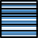Profiles taken in the fast scanning axis (usually x-axis) can be mutually shifted by some amount or have slightly different slopes. The basic line correction function Data ProcessCorrect DataAlign Rows deals with this type of discrepancy using several different correction algorithms:

Median

A basic correction method, based on finding a representative height of each scan line and subtracting it, thus moving the lines to the same height. Here the line median is used as the representative height.

Modus

This method differs from Median only in the quantity used: modus of the height distribution. Of course, the modus is only estimated because only a finite set of heights is avaiable.

Polynomial

The Polynomial method fits a polynomial of given degree and subtracts if from the line. For polynomial degree of 0 the mean value of each row is subtracted. Degree 1 means removal of linear slopes, degree 2 bow removal, etc.

Median difference

In contrast to shifting a representative height for each line, Median difference shifts the lines so that the median of height differences (between vertical neighbour pixels) becomes zero. Therefore it better preserves large features while it is more sensitive to completely bogus lines.

Matching

This algorithm is somewhat experimental but it may be useful sometimes. It minimizes a certain line difference function that gives more weight to flat areas and less weight to areas with large slopes.

Trimmed mean

Trimmed mean lies between the standard mean value and median, depending on how large fraction of lowest and highest values are trimmed. For no trimming (0) this method is equivalent to mean value subtraction, i.e. Polynomial with degree 0, for maximum possible trimming (0.5) it is equivalent to Median.

Trimmed mean of differences

This method similarly offers a continous transition between Median difference and mean value subtraction. It makes zero the trimmed means of height differences (between vertical neighbour pixels). For the maximum possible trimming (0.5) it is equivalent to Median difference. Since the mean difference is the same as the difference of mean values (unlike for medians), for no trimming (0) it is again equivalent to Polynomial with degree 0.

Similarly as in the two-dimensional polynomial levelling, the background, i.e. values subtracted from individual rows can be extracted to another image. Or plotted in a graph since the value is the same for the entire row.

The line correction function support masking, allowing the exclusion of large features that could distract the corection algorithms. The masking options are offered only if a mask is present though. Note the Path level tools described below offers a different method of choosing the image parts important for alignment. It can be more convenient in some cases.

### Tip

Use Ctrl-F (Repeat Last) to run the line correction with the same settings on several images without going through the dialogue.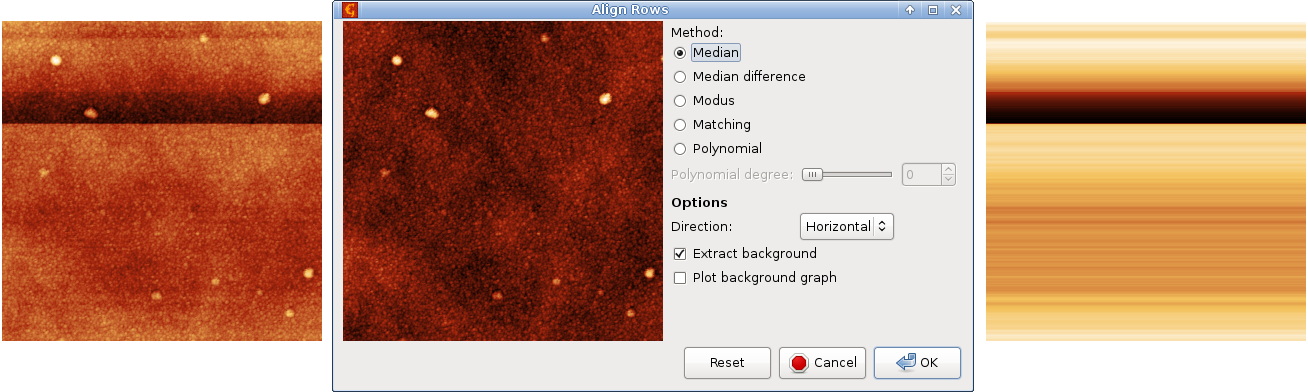Line correction example: an image with defects (left), the row alignment dialogue previewing the median correction (centre), and extracted row background (right). Note the false colour scales are not the same in the three images.

### Path Leveling Tool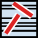The Path Leveling tool can be used to correct the heights in an arbitrary subset of rows in complicated images.

First, one selects a number of straight lines on the data. The intersections of these lines with the rows then form a set of points in each row that is used for leveling. The rows are moved up or down to minimize the difference between the heights of the points of adjacent rows. Rows that are not intersected by any line are not moved (relatively to neighbouring rows).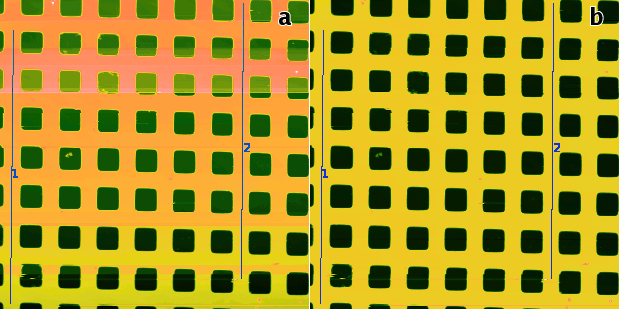Path Level example: (a) uncorrected data with steps that the automated method may fail to correct, two suitable leveling lines are selected; (b) the result of Path Level application with line width 5.

### Step Line Correction

Function Step Line Correction attempts to deal with shifts that may occur in the middle of a scan line. It tries to identify misaligned segments within the rows and correct the height of each such segment individually. Therefore it is often able to correct data with discontinuities in the middle of a row. This function is somewhat experimental and the exact way it works can be subject to futher changes.

### Mark Inverted Rows

Function Mark Inverted Rows finds scan lines with vertically inverted features and marks them with a mask. Line inversion is an artefact which occasionally occurs for instance in Magnetic Force Microscopy. Since the line is generally only inverted very approximately, value inversion would be a poor correction and one should usually use Laplace's interpolation for correction.

### Mark Scars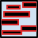Data ProcessCorrect DataMark Scars

Similarly, the `Mark Scars` module can create a mask of the points treated as scars. Unlike Remove Scars which directly interpolates the located defects, this module lets you interactively set several parameters which can fine-tune the scar selection process:

• Maximum width – only scars that are as thin or thinner than this value (in pixels) will be marked.
• Minimum length – only scars that are as long or longer than this value (in pixels) will be marked.
• Hard threshold – the minimum difference of the value from the neighbouring upper and lower lines to be considered a defect. The units are relative to image RMS.
• Soft threshold – values differing at least this much do not form defects themselves, but they are attached to defects obtained from the hard threshold if they touch one.
• Positive, Negative, Both – the type of defects to remove. Positive means defects with outlying values above the normal values (peaks), negative means defects with outlying values below the normal values (holes).

After clicking the new scar mask will be applied to the image. Other modules or tools can then be run to edit this data.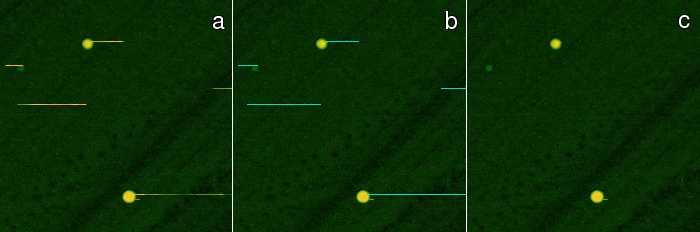Scars marking and removal example: (a) original data with defects, (b) data with marked deffects, (c) corrected data.

### Remove Scars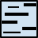Data ProcessCorrect DataRemove Scars

Scars (or stripes, strokes) are parts of the image that are corrupted by a very common scanning error: local fault of the closed loop. Line defects are usually parallel to the fast scanning axis in the image. This function will automatically find and remove these scars, using neighbourhood lines to fill-in the gaps. The method is run with the last settings used in Mark Scars.

### XY denoising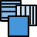Data ProcessMultidataXY denoise

The function denoises and image on the basis of two measurements of the same area – one performed in x direction and one in y direction (and rotated back to be aligned the same way as the x-direction one). It is based on work of E. Anguiano and M. Aguilar (see ).

The denoising works by performing the Fourier transform of both images, combining the information them in the frequency space, and then using backward Fourier transform in order to get the denoised image. It is useful namely for the removal of large scars and fast scanning axis stripes.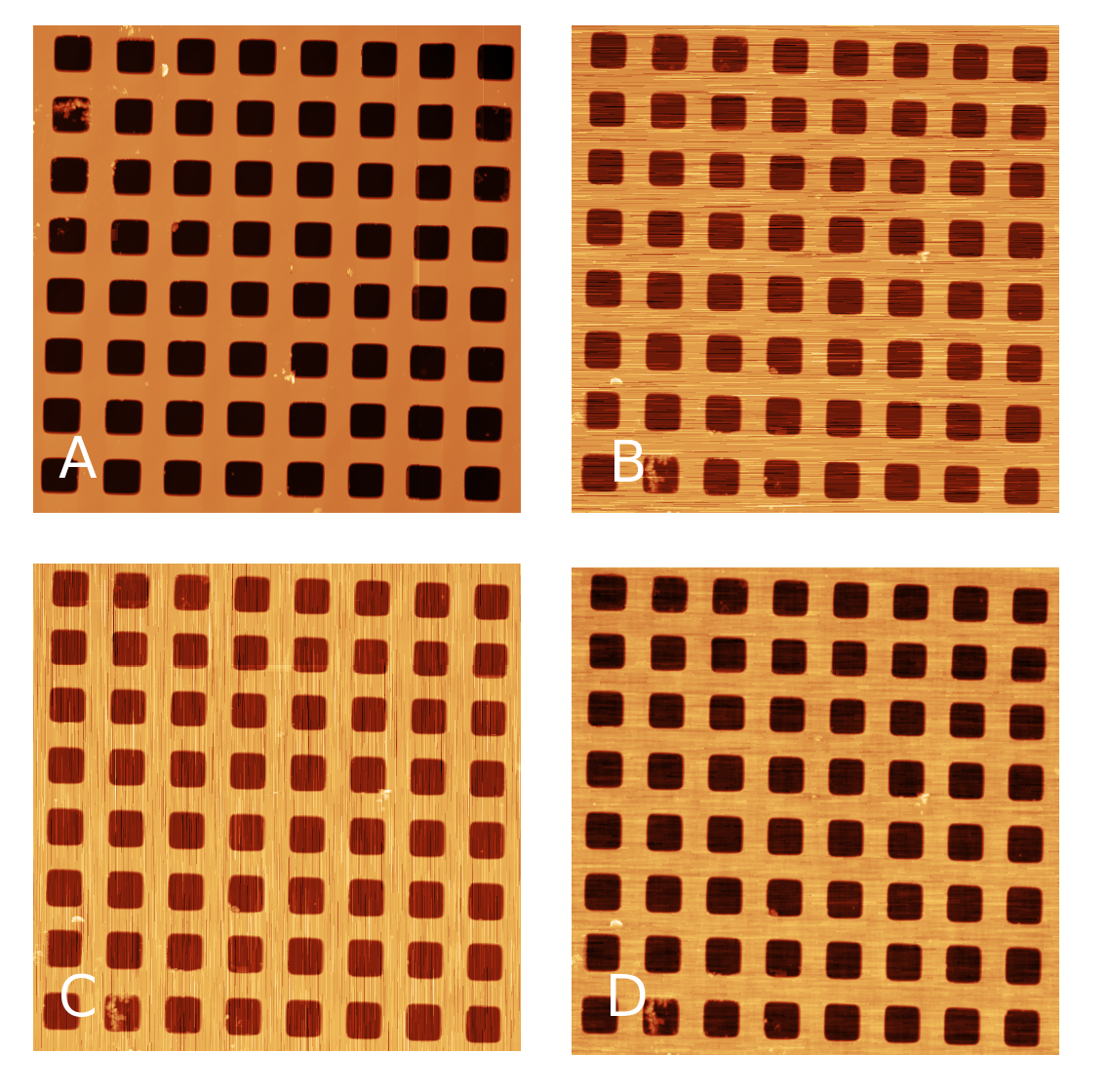XY denoise procedure simulation: A) original data, B) simulated measurement in x axis, C) simulated measurement in y axis, D) denoised image.